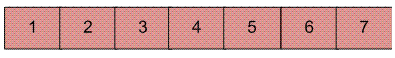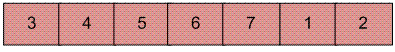# Block swap algorithm for array rotation

Write a function rotate(ar[], d, n) that rotates arr[] of size n by d elements.Rotation of the above array by 2 will make array## Recommended: Please try your approach on {IDE} first, before moving on to the solution.

Algorithm :

```Initialize A = arr[0..d-1] and B = arr[d..n-1]
1) Do following until size of A is equal to size of B

a)  If A is shorter, divide B into Bl and Br such that Br is of same
length as A. Swap A and Br to change ABlBr into BrBlA. Now A
is at its final place, so recur on pieces of B.

b)  If A is longer, divide A into Al and Ar such that Al is of same
length as B Swap Al and B to change AlArB into BArAl. Now B
is at its final place, so recur on pieces of A.

2)  Finally when A and B are of equal size, block swap them.
```

Recursive Implementation:

## C++

 `#include ` `using` `namespace` `std; ` ` `  `/*Prototype for utility functions */` `void` `printArray(``int` `arr[], ``int` `size);  ` `void` `swap(``int` `arr[], ``int` `fi, ``int` `si, ``int` `d);  ` ` `  `void` `leftRotate(``int` `arr[], ``int` `d, ``int` `n)  ` `{  ` `    ``/* Return If number of elements to be rotated   ` `    ``is zero or equal to array size */` `    ``if``(d == 0 || d == n)  ` `        ``return``;  ` `         `  `    ``/*If number of elements to be rotated  ` `    ``is exactly half of array size */` `    ``if``(n - d == d)  ` `    ``{  ` `        ``swap(arr, 0, n - d, d);  ` `        ``return``;  ` `    ``}  ` `         `  `    ``/* If A is shorter*/`         `    ``if``(d < n - d)  ` `    ``{  ` `        ``swap(arr, 0, n - d, d);  ` `        ``leftRotate(arr, d, n - d);      ` `    ``}  ` `    ``else` `/* If B is shorter*/`         `    ``{  ` `        ``swap(arr, 0, d, n - d);  ` `        ``leftRotate(arr + n - d, 2 * d - n, d); ``/*This is tricky*/` `    ``}  ` `}  ` ` `  `/*UTILITY FUNCTIONS*/` `/* function to print an array */` `void` `printArray(``int` `arr[], ``int` `size)  ` `{  ` `    ``int` `i;  ` `    ``for``(i = 0; i < size; i++)  ` `        ``cout << arr[i] << ``" "``;  ` `    ``cout << endl;  ` `}  ` ` `  `/*This function swaps d elements starting at index fi  ` `with d elements starting at index si */` `void` `swap(``int` `arr[], ``int` `fi, ``int` `si, ``int` `d)  ` `{  ` `    ``int` `i, temp;  ` `    ``for``(i = 0; i < d; i++)  ` `    ``{  ` `        ``temp = arr[fi + i];  ` `        ``arr[fi + i] = arr[si + i];  ` `        ``arr[si + i] = temp;  ` `    ``}  ` `}  ` ` `  `// Driver Code ` `int` `main()  ` `{  ` `    ``int` `arr[] = {1, 2, 3, 4, 5, 6, 7};  ` `    ``leftRotate(arr, 2, 7);  ` `    ``printArray(arr, 7);  ` `    ``return` `0;  ` `}  ` ` `  `// This code is contributed by rathbhupendra `

## C

 `#include ` ` `  `/*Prototype for utility functions */` `void` `printArray(``int` `arr[], ``int` `size); ` `void` `swap(``int` `arr[], ``int` `fi, ``int` `si, ``int` `d); ` ` `  `void` `leftRotate(``int` `arr[], ``int` `d, ``int` `n) ` `{  ` `  ``/* Return If number of elements to be rotated is  ` `    ``zero or equal to array size */`   `  ``if``(d == 0 || d == n) ` `    ``return``; ` `     `  `  ``/*If number of elements to be rotated is exactly  ` `    ``half of array size */`   `  ``if``(n-d == d) ` `  ``{ ` `    ``swap(arr, 0, n-d, d);    ` `    ``return``; ` `  ``}   ` `     `  ` ``/* If A is shorter*/`               `  ``if``(d < n-d) ` `  ``{   ` `    ``swap(arr, 0, n-d, d); ` `    ``leftRotate(arr, d, n-d);     ` `  ``}     ` `  ``else` `/* If B is shorter*/`               `  ``{ ` `    ``swap(arr, 0, d, n-d); ` `    ``leftRotate(arr+n-d, 2*d-n, d); ``/*This is tricky*/` `  ``} ` `} ` ` `  `/*UTILITY FUNCTIONS*/` `/* function to print an array */` `void` `printArray(``int` `arr[], ``int` `size) ` `{ ` `  ``int` `i; ` `  ``for``(i = 0; i < size; i++) ` `    ``printf``(``"%d "``, arr[i]); ` `  ``printf``(``"\n "``); ` `}  ` ` `  `/*This function swaps d elements starting at index fi ` `  ``with d elements starting at index si */` `void` `swap(``int` `arr[], ``int` `fi, ``int` `si, ``int` `d) ` `{ ` `   ``int` `i, temp; ` `   ``for``(i = 0; i

## Java

 `import` `java.util.*; ` ` `  `class` `GFG  ` `{ ` `    ``// Wrapper over the recursive function leftRotateRec() ` `    ``// It left rotates arr[] by d. ` `    ``public` `static` `void` `leftRotate(``int` `arr[], ``int` `d,  ` `                                                ``int` `n) ` `    ``{ ` `        ``leftRotateRec(arr, ``0``, d, n); ` `    ``} ` ` `  `    ``public` `static` `void` `leftRotateRec(``int` `arr[], ``int` `i,  ` `                                  ``int` `d, ``int` `n) ` `    ``{ ` `        ``/* Return If number of elements to be rotated  ` `        ``is zero or equal to array size */` `        ``if``(d == ``0` `|| d == n)  ` `            ``return``;  ` `         `  `        ``/*If number of elements to be rotated  ` `        ``is exactly half of array size */` `        ``if``(n - d == d)  ` `        ``{  ` `            ``swap(arr, i, n - d + i, d);  ` `            ``return``;  ` `        ``}  ` `         `  `        ``/* If A is shorter*/`     `        ``if``(d < n - d)  ` `        ``{  ` `            ``swap(arr, i, n - d + i, d);  ` `            ``leftRotateRec(arr, i, d, n - d);      ` `        ``}  ` `        ``else` `/* If B is shorter*/`     `        ``{  ` `            ``swap(arr, i, d, n - d);  ` `            ``leftRotateRec(arr, n - d + i, ``2` `* d - n, d); ``/*This is tricky*/` `        ``}  ` `    ``} ` ` `  `/*UTILITY FUNCTIONS*/` `/* function to print an array */` `public` `static` `void` `printArray(``int` `arr[], ``int` `size)  ` `{  ` `    ``int` `i;  ` `    ``for``(i = ``0``; i < size; i++)  ` `        ``System.out.print(arr[i] + ``" "``);  ` `    ``System.out.println();  ` `}  ` ` `  `/*This function swaps d elements  ` `starting at index fi with d elements ` `starting at index si */` `public` `static` `void` `swap(``int` `arr[], ``int` `fi, ` `                        ``int` `si, ``int` `d)  ` `{  ` `    ``int` `i, temp;  ` `    ``for``(i = ``0``; i < d; i++)  ` `    ``{  ` `        ``temp = arr[fi + i];  ` `        ``arr[fi + i] = arr[si + i];  ` `        ``arr[si + i] = temp;  ` `    ``}  ` `}  ` ` `  `// Driver Code ` `public` `static` `void` `main (String[] args)  ` `{ ` `    ``int` `arr[] = {``1``, ``2``, ``3``, ``4``, ``5``, ``6``, ``7``};  ` `    ``leftRotate(arr, ``2``, ``7``);  ` `    ``printArray(arr, ``7``);      ` `} ` `} ` ` `  `// This code is contributed by codeseeker `

Iterative Implementation:
Here is iterative implementation of the same algorithm. Same utility function swap() is used here.

## C

 `void` `leftRotate(``int` `arr[], ``int` `d, ``int` `n) ` `{ ` `  ``int` `i, j; ` `  ``if``(d == 0 || d == n) ` `    ``return``; ` `  ``i = d; ` `  ``j = n - d; ` `  ``while` `(i != j) ` `  ``{ ` `    ``if``(i < j) ``/*A is shorter*/` `    ``{ ` `      ``swap(arr, d-i, d+j-i, i); ` `      ``j -= i; ` `    ``} ` `    ``else` `/*B is shorter*/` `    ``{ ` `      ``swap(arr, d-i, d, j); ` `      ``i -= j; ` `    ``} ` `    ``// printArray(arr, 7); ` `  ``} ` `  ``/*Finally, block swap A and B*/` `  ``swap(arr, d-i, d, i); ` `} `

## Java

 `//Java code for above implementation ` `static` `void` `leftRotate(``int` `arr[], ``int` `d, ``int` `n)  ` `{  ` `int` `i, j;  ` `if``(d == ``0` `|| d == n)  ` `    ``return``;  ` `i = d;  ` `j = n - d;  ` `while` `(i != j)  ` `{  ` `    ``if``(i < j) ``/*A is shorter*/` `    ``{  ` `    ``swap(arr, d-i, d+j-i, i);  ` `    ``j -= i;  ` `    ``}  ` `    ``else` `/*B is shorter*/` `    ``{  ` `    ``swap(arr, d-i, d, j);  ` `    ``i -= j;  ` `    ``}  ` `    ``// printArray(arr, 7);  ` `}  ` `/*Finally, block swap A and B*/` `swap(arr, d-i, d, i);  ` `}  `

## Python3

 `# Python3 code for above implementation ` ` `  `def` `leftRotate(arr, d, n):  ` `    ``if``(d ``=``=` `0` `or` `d ``=``=` `n):  ` `        ``return``;  ` `    ``i ``=` `d  ` `    ``j ``=` `n ``-` `d  ` `    ``while` `(i !``=` `j):  ` `         `  `        ``if``(i < j): ``# A is shorter ` `            ``swap(arr, d ``-` `i, d ``+` `j ``-` `i, i)  ` `            ``j ``-``=` `i  ` `         `  `        ``else``: ``# B is shorter ` `            ``swap(arr, d ``-` `i, d, j)  ` `            ``i ``-``=` `j  ` `     `  `    ``swap(arr, d ``-` `i, d, i) ` ` `  `# This code is contributed  ` `# by Adarsh_Verma `

## C#

 `// C# code for above implementation  ` `static` `void` `leftRotate(``int` `[]arr, ``int` `d, ``int` `n)  ` `{  ` `    ``int` `i, j;  ` `    ``if``(d == 0 || d == n)  ` `        ``return``;  ` `    ``i = d;  ` `    ``j = n - d;  ` `    ``while` `(i != j)  ` `    ``{  ` `        ``if``(i < j) ``/*A is shorter*/` `        ``{  ` `            ``swap(arr, d-i, d+j-i, i);  ` `            ``j -= i;  ` `        ``}  ` `        ``else` `/*B is shorter*/` `        ``{  ` `            ``swap(arr, d-i, d, j);  ` `            ``i -= j;  ` `        ``}  ` `         `  `    ``}  ` `     `  `    ``/*Finally, block swap A and B*/` `    ``swap(arr, d-i, d, i);  ` `}  ` ` `  `// This code is contributed by Rajput-Ji `

Time Complexity: O(n)

Please see following posts for other methods of array rotation:
https://www.geeksforgeeks.org/array-rotation/
https://www.geeksforgeeks.org/program-for-array-rotation-continued-reversal-algorithm/

Please write comments if you find any bug in the above programs/algorithms or want to share any additional information about the block swap algorithm.

Don’t stop now and take your learning to the next level. Learn all the important concepts of Data Structures and Algorithms with the help of the most trusted course: DSA Self Paced. Become industry ready at a student-friendly price.

My Personal Notes arrow_drop_up

Article Tags :
Practice Tags :

43

Please write to us at contribute@geeksforgeeks.org to report any issue with the above content.